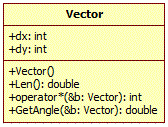Competitions

# Vector. Scalar Product

You are given two vectors. Find their scalar product and the angle between them.

Write the code according to the next interface:

class Vector // C++
{
public:
int dx, dy;
Vector(void); // Constructor. Read the vector coordinates
double Len(void); // Return the length of a vector
int operator *(Vector &b); // Overload operator *: return the scalar product
double GetAngle(Vector &b); // Return in radians the angle between current vector and vector b
};class Vector // Java
{
private int dx, dy;
Vector(); // Constructor, creates vector (0, 0)
Vector(int dx, int dy); // Constructor, creates vector (dx, dy)
public double getLength(void); // Returns the length of the vector
public int Scalar(Vector v); // Returns the scalar product of current vector and vector v
public double GetAngle(Vector v); // Returns in radians the angle between current vector and vector v
};


#### Input

Four integers - the coordinates of two nonzero vectors. All values do not exceed 10000 by the absolute value.

#### Output

In the first line print the scalar product of the vectors and in the second line print the value of an undirected angle between them up to the fifth decimal place in the interval [0; π].

Time limit 1 second
Memory limit 128 MiB
Input example #1
2 1 3 5

Output example #1
11
0.56673

Author Mykhailo Medvediev
Source C++ Language